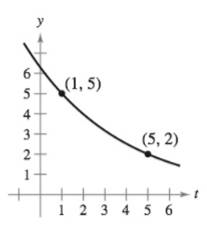Chapter 6.2, Problem 25E

Chapter
Section
Textbook Problem

Finding an Exponential Function InExercises 23-26, find the exponential function y = C e k t that passes through the two given points.To determine

To calculate: Exponential function y=Cekt that is passing through the two points (1,5) and (5,2).

Explanation

Given:

The given figure is:

Exponential function is y=Cekt.

Formula used:

Logarithmic property:

ln(ex)=x

And,

eln(x)=x

Calculation:

Let us consider the given function y=Cekt.

And, the two points are (1,5) and (5,2).

By putting the first point (1,5) in the given function, we get:

y=Cekt5=Cek×1Cek=5C=5ek

Next, by putting the second point (5,2) and C=5ek in the given equation, we get:

y=Cekt2=5ek×ek×52=5e5kk2=5e4

Still sussing out bartleby?

Check out a sample textbook solution.

See a sample solution

The Solution to Your Study Problems

Bartleby provides explanations to thousands of textbook problems written by our experts, many with advanced degrees!

Get Started